# MP Board Class 11th Chemistry Important Questions Chapter 4 Chemical Bonding and Molecular Structure

## MP Board Class 11th Chemistry Important Questions Chapter 4 Chemical Bonding and Molecular Structure

### Chemical Bonding and Molecular Structure Important Questions

Chemical Bonding And Molecular Structure Very Short Answer Type Questions

Question 1.
What type of bond is present generally in same atoms?
Covalent bond.

Question 2.
Which hybridization is present in ammonia (NH3)?
sp3.

Question 3.
What is the reason for high boiling point of water?
Presence of H – bond between molecules of water.

Question 4.
What is the dipole moment of C02?
Zero.Question 5.
What type of bonds are directional?
Covalent bonds.

Question 6.
What is the bond angle in water?
104°5′.

Question 7.
What is the structure of [Ni(CN)4]2-?
Square planner.

Question 8.
Which bond is present in s – s overlapping?
cr (Sigma) bond.

Question 9.
What is the structure of diamond?
Crystal lattice (Tetrahedral).

Question 10.
Which bond is present in sidewise overlapping of p – p orbitals?
n – bond.Question 11.
Which type of hybridization found in PCl5?
sjy’d hybridization.

Question 12.
What is full form of LCAO?
Linear Combination of Atomic Orbitals.

Question 13.
What is the structure of NH3?
Trigonal bipyramidal.

Question 14.
What is dipole moment (µ) of a linear covalent molecule?
Zero.

Question 15.
What is the unit of electron gain enthalpy?
eV per atom or kJ per mole.

Question 16.
What is the formula of bond order?
Bond order = $$\frac{1}{2}$$ (Nb – Na).

Question 17.
What is the symbol of superoxide and peroxide?
Superoxide – O2 and peroxide – O2-2.

Question 18.
What do you mean by bond order?
The number of electrons present between two atoms of molecules or ions.

Question 19.
More polarizing power and more polarisability increases which property of molecule?
Covalent property.Question 20.
What is determined by Born – Haber cycle?
Lattice energy.

Question 21.
What is full form of VSEPR?
Valence Shell Electron Pair Repulsion.

Chemical Bonding And Molecular Structure Short Answer Type Questions – I

Question 1.
What is the electronic theory of covalency? Write its main postulates?
The electronic theory of valency and its main postulates are as follows:

1. The covalency of any element depends upon the no.of electrons present in its valence shell.
2. All the elements have the tendency to acquire the nobel gas configuration.
3. The electrons present in valence shell are called valence electrons and when the electron comes out than the vacant space is called kernel.
4. If an element is unstable, than it works for its stability for this it gives and takes the electron. On this basis the bonds are of three types:
1. Ionic bond
2. Covalent bond and
3. Co – ordinate bond.

Question 2.
What do you mean by lone pair of electron?
The electron pair which present in the valence orbital of the element and does not take part bond formation is called lone pair electron.
Example: In the H2O atom the lone pairs of electrons on an atom: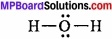Bond pairs = 2, Lone pair electrons = 2.

Question 3.
What do you mean by dipole moment?
Dipole moment is defined as, the product ofthe magnitude of charge on any one of the atoms and distance between them. It is represented by Greek letter µ(mu).
Mathematically, dipole moment is expressed as µ = e × d
Where, e is charge on any one of the atoms and d is distance between the atoms.
As e is of the order of 10-10 esu while d is of the order of 10-8 cm µ is of the order 1018 esu cm and this unit of p is known as Debye (D). Thus,
1D = 1 × 10-18esu cm

Question 4.
Is He2 molecule is possible? Clearify it.
He2 molecule is not possible.
2He → 1s2
Two electrons are present in Is orbital of He atom. It is complete and stable orbital and so it cannot accept an extra electron. The bond order is zero. So the formation of He2 molecule is impossible.

Question 5.
What is the total number of σ (sigma) and π (pi) bond are present in following molecules:

1. C2H2
2. C2H4

1. C2H2
2. C2H4Question 6.
What do you mean by hydrogen bond?
Hydrogen bond is defined as the electrostatic force of attraction which exists between the covalently bonded hydrogen atom of one molecule and the electronegative atom of the other molecule. Hydrogen bond is of two types:

1. Intermolecular hydrogen bonding
2. Intra – molecular hydrogen bonding.

Question 7.
At normal temperature H20 is in liquid state but H2S is in gaseous state. Why?
Hydrogen bonding affects the physical state of the molecule. For example, H20 and H2S are the hydrides of group 16 elements, but H2O is liquid whereas H2S is gas at room temperature. Actually, H2O fulfils the (MPBoardSolutions.com) condition of hydrogen bonding therefore, in H2O hydrogen bonding is found and they get associated, grow in molecular size and exist in liquid state. The H2S molecule, the magnitude of hydrogen bonding is negligible, therefore, molecules remains separated from each other and acquires gaseous state at room temperature.Question 8.
Why, viscosity of glycerol is more than ethanol?
In ethanol molecule, one hydroxyl gap is present whereas in every glycerol, three OH – groups are present which form hydrogen bond. Therefore, in glycerol hydrogen bond formation is more in comparison to ethanol. That is why, the viscosity of glycerol is more than ethanol.

Question 9.
Why σ – bond is stronger than π – bond?
The strength of any bond depends upon the overlapping limit, σ – bonds are formed by axial overlapping whereas σ – bonds are formed by sidewise overlapping. So, the extent of overlapping in σ – bond is more than π – bond. So σ – bond is more stronger than π – bond.

Question 10.
Why HF molecule is more polar than HI?
The electronegativity of F is more than I. So, the displacement of electron in covalency in HF is more than HI, resultant the deviation of charges in HF is greater than HI. So, HF is more polar than HI.

Question 11.
C – Cl bond is polar but CCl4 is non – polar, why? Give the reason?
In C – Cl bond the electronegativity of Cl is more than C, due to this the electrons in covalent bond shifts towards Cl, due to which partial +ve charge appears on C and partial -ve charge develops once and this bond become polar in nature. Whereas the structure of CCl4 is symmetric, due to which the dipole moment of C – Cl bond cancelled each other. So, CCl4 molecule is non – polar.

Question 12.
What do you mean by resonance?
Some compounds cannot be represented by a single definite structure, rather more than one structure is required by none of them is able to explain all the known properties of the compound alone. Thus, the various structures written for a compound to explain the known properties of it completely are called resonating structure. This phenomenon is called resonance.

Question 13.
What are the conditions for resonance?
The conditions are as follows:

1. The heat of formation of each resonating structure should be same.
2. The arrangement of atoms in each formula should be same.
3. The number of unpaired electron in each structures should be same.

Question 14.
What is resonance energy ?
The difference between the actual energy of the resonance hybrid and the most stable one of the resonating structures is called resonance energy.Question 15.
Determine the bond order in N2?
N2 (14 electrons): The electronic configuration
σ1s2, σ*1s2, σ2s2, σ*2s2, (π2px2 = π2py2), σ2pz2
Bond order = $$\frac{1}{2}$$ (Nb – Na) = $$\frac{1}{2}$$ × (10 – 4) = 3.

Question 16.
On the basis of molecular orbital theory explain that the Be2 atom is not formed?
4Be: Electronic configuration = 1s2 2s2
Be2 (molecule) (4 + 4 = 8e)
Electronic configuration = σ1s2, σ*1s2, σ2s2, σ*2s2
Bond order = $$\frac{1}{2}$$ (Nb – Na) = $$\frac{1}{2}$$ × (4 – 4) = 0.
So, the Be2 molecule does not formed.

Question 17.
Write the definition of hybridization?
The process of intermixing of atomic orbitals of nearly equal energy and proper symmetry giving rise to equal number of new orbitals of same energy is called hybridization and the orbitals so formed hybridized orbital.

Question 18.
What do you understand by lattice energy and solvation energy?
Lattice energy:
Once the gaseous ions are formed, the ions of opposite charges come close together and pack up three – dimensionally in a definite geometric pattern to form ionic crystal (Crystal lattice). Since, the packing of ions of opposite charges takes place as a result of attractive force between them, the process is accompanied (MPBoardSolutions.com) with the release of energy referred to as lattice enthalpy. Lattice enthalpy may be defined as; the amount of energy released when one mole of ionic solid is formed by the close packing of its constituents. It is denoted by ∆LH and negative in nature.

Solvation energy:
The energy released when an ion get soluble in water is called solvation energy.Question 19.
Whose boiling points are high electrovalent compounds or covalent compounds, why?
High boiling points: The boiling points of ionic solids are very high. This is due to strong electrostatic force of attraction between the oppositely charged ions. To change the physical state of ionic compounds, high temperature is required.

Question 20.
Why BaS04 is insoluble in water?
The solubility of any ionic compound depends upon the lattice energy and solvation energy. If the lattice energy of any compound is more than solvation energy, than ionic compound is insoluble in water. The solvation energy of BaS04 is less than lattice energy. So it is insoluble in water.

Question 21.
If Be – H bond is polar, the dipole moment of Be – H2 is zero. Why?
BeH2 is linear. The bond moment present in opposite direction cancelled each other. That is why Be the dipole moment of BeH2 is zero.Chemical Bonding And Molecular Structure Short Answer Type Questions – II

Question 1.
Why ice is lighter than water? Explain?
Or, Density of ice is less than water. Why?
Density of ice is less than water:
In ice each oxygen atom is tetrahedrally surrounded by four hydrogen atoms in which two hydrogen atoms are linked to oxgyen atom by covalent bond and other two hydrogen atoms are linked by hydrogen bond.The molecules of H2O are not packed closely. This H gives rise to open cage like structure for ice having a larger volume for the given mass of water. Thus, density of ice is less than water. Ice is actually hydrogen bonded crystal. (MPBoardSolutions.com) Three dimensional structure of protein and nucleic acids like biologically important substances is due to hydrogen bond. Energy of hydrogen bond is between 3.5 kJmol-1 and 8 kJmol-1. Thus, hydrogen bond is stronger than van der Waals force and weaker than covalent bond.

Question 2.
Write the rules of hybridization?
Conditions for hybridization: Following are the conditions for hybridization:

1. The orbitals of one and same atom participate in hybridization. Only the orbitals and not electrons get hybridized.
2. The energy difference between the hybridizing orbitals should be small.
3. Promotion of electron is not essential prior to hybridization.
4. It is not necessary that only the half-filled orbitals may participate in hybridiza¬tion. In some cases, even the filled orbitals may participate in hybridization.Question 3.
Explain covalent bond with example?
Lewis – Langmuir theory:
Lewis and Langmuir suggested that, atoms may combine by sharing of electrons in their outermost shell to complete their respective octet. The shared electrons becomes the property of both the atoms. This types of linkage is known as covalent linkage or covalent bond. Thus,

1. The force which binds atoms of same or different elements by mutual sharing of electrons is called a covalent bond.

2. This type of bond is formed between two similar non-metalic elements (A, A) or (B, B) or dissimilar atoms (A and B).
Example:
Chlorine molecule:
Both the chlorine atoms (Z = 17) contain 7 electrons in their valence shells and short in one electron each. They share one electron pair in which an electron is contributed by both as shown below:Question 4.
Differentiate between atom and ion?
Differences between Atom and Ion:
Atom:

1. Atoms are electroneutral.
2. Not present in free state.
3. Takes part in chemical reaction.
4. No. of e and proton is same.

Ion:

1. Ions are charged.
2. Ions present in free state.
3. Not take part in chemical reaction.
4. No. of e is more than proton.

Question 5.
Differentiate between Sigma (σ) and Pi (π) bond?
Differences between Sigma (σ) and Pi (π) bond:
Sigma (σ) bond:

1. This bond is formed by end to end or head on overlapping of orbitals along the inter nuclear axis.
2. This is formed by overlapping of s – s, s – p or p – p orbitals.
3. Overlapping is large, hence it is strong bond.
4. Free rotation about σ – bond is possible.
5. Electron cloud is symmetrical about inter nuclear axis.

Pi (π) bond:

1. This bond is formed by the sidewise overlapping of orbitals.
2. This is formed by overlapping of p – p orbitals only.
3. Overlapping is small, hence it is weak bond.
4. Free rotation about a π – bond is not possible.
5. Electron cloud of π – bond is unsymmetrical.Question 6.
Write difference between s – and p – orbitals?
Differences between s – and p – orbitals:
s – orbital:

1. They are oval – symmetrical.
2. Non – directional
3. 1 = 0 and m = 0

p – orbitals:

1. They are dumbelled shape and lines symmetry of axis.
2. Directional
3. l = 1 and m = -1, 0, +1

Question 7.
Explain inter molecular and intramolecular hydrogen bonds with example?
Types of hydrogen bond:
1. Intermolecular hydrogen bonding:
When these atoms (hydrogen and electronegative atom) are of different molecules, it is called intermolecular hydrogen bonding as in H2O, HF, C2H5OH etc.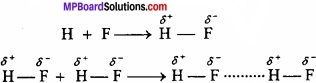Hydrogen bond is represented by dotted lines. Many molecules of HF associates and form (HF)n. On the same way molecules of water and alcohols are linked with hydrogen bonds.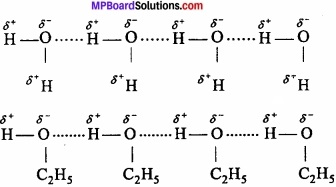2. Intramolecular hydrogen bonding:
If these atoms (hydrogen and electronegative atoms) are present in same molecule, this type of hydrogen bonding is called intramolecular hydrogen bonding.
e.g., o – nitrophenolQuestion 8.
Among NH3 and NF3 whose dipole moment is high. Why?
Both these molecules have pyramidal shape with one lone pair of electron on nitrogen atom. As fluorine is more electronegative than hydrogen therefore N – F bond should be more polar than N – H bonds. Consequently, the resultant dipole moment of NF3 should be much larger than that of NH3. However, the dipole moment of NH3 (µ = 1.47D) is larger than that of NF3 (µ = 0.24D).

The anomalous behaviour can be explained due to the presence of lone pair on nitrogen. In case of NH3, the orbital dipole due to lone pair of electron and the bond moments of three N – H bonds are in same direction. Therefore, it adds on the resultant dipole moment of the N – H bonds. On the other hand in case of NF3, the orbital dipole moment is in the opposite direction to resultant dipole moment of three N – F bonds. Thus, the lone pair moment cancels the resultant N – F bond moments as shown in figure. Consequently, the dipole moment of NF3 is low.

Question 9.
Is according to following equation, is the hybridization changes in B and N:
BF3 + NH3 → F3B.NH3.
In BF3 three bonded pair and zero lone pair electrons are present. Due to this B is sp2 hybridized and in NH3 three bonded pair and one lone pair of electron is present. So, N is sp3 hybridized. After reaction the hybridization of B becomes sp3 but the hybridization of N remains same as N gives its lone pair to B atom.

Question 10.
Explain the change in hybridization in A1 atom in following reaction:
AlCl3 + cl → AlCl4
The electronic configuration of Al is:
At ground state = 13Al = ls2 2s2 2p6 3s2 3px1
At excited state = 1s2, 2s2, 2p6 3s2 3px1 y1
In the formation of AlCl3, Al is sp2 hybridized and its geometry is trigonal bipyramidal. Whereas in the formation of AlCl4, due to inclusion of 3pz orbital. Al is sp3 hybridized and its geometry is tetrahedral.Question 11.
What are the postulates of orbital overlap concept of covalent bond?
According to this concept:
1. The covalent bond is formed due to partial overlap of the two half-filled atomic orbitals of the valence shells of the combining atoms. Partial overlap means that a part of the electron cloud of each of the two half – filled domic orbitals becomes common. As a result the probability of finding electrons in the region of overlap is much more at the other places. This reduces the intemuclear repulsion and hence decreases the energy.

2. The orbitals undergoing overlap must have electrons with opposite spins.

3. Greater the extent of overlapping, stronger is the bond formed.

4. Larger the size of the orbitals, less effective is the overlapping and thus weaker is the bond formed.

Question 12.
Explain that the geometry of PCl5 is trigonal bipyramidal and that of IF5 is pyramidal?
PCl5: P is central metal atom.As P in PCl5 in sp3d hybridized so its geometry is square pyramidal.
IF5: Central Metal atom is I (Z = 53)In IF7 , I is sp3d2

Question 13.
What are σ bond and π bond? Explain with example?
Hybridization is defined as, “The process of intermixing of atomic orbitals of nearly equal energy and proper symmetry giving rise to equal number of new orbitals of same energy is called hybridization and the orbitals so formed hybridized orbitals.”

Sigma bond:
The bond formed by overlapping of two orbitals along their axis is called a sigma (σ) bond. The line joining the two nuclei of the combining atoms is called the intemuclear axis or bond axis.
Example: This type of overlapping takes place between s – s orbital, s – p orbital and pz – pz orbitals.Pi – bond:
The bond formed by the lateral overlapping of two p – orbitals (px – px) (py – py) is called π – bond.It is important to note that overlapping at both the lobes of the p – orbital occurs in Pi – bond whereas in case of sigma bond the overlap occurs in a single region.

Question 14.
Give the reason for the difference in properties of two allotropes diamond and graphite of carbon?
Diamond and graphite are two allotropes of carbon. But due to difference in C arrangement their properties are different. In diamond the C atom is sp3 hybridized. Every C atom attached with four cations form tetrahedral geometry. So it forms a lattice structure and so hard and have high melting point.

In graphite every C atom is sp2 hybridized, i.e. each C is surrounded by three cations and fourth valency of C is unstable. In graphite different layers are present which are joined together with weak vander Waals’ forces. That is why graphite is soft and due to presence of free electron it conducts electricity.Question 15.
Explain the hybridized structure of acetylene by diagram?
Formation of ethyne or acetylene (HC = CH): In the formation of acetylene molecule, each carbon atom undergoes ip – hybridization leaving two 2p – orbitals in the original unhybridized state. The two sp – hybrid orbitals of carbon atom are linear and are directed at an angle of 180°. Whereas the two unhybridized p – orbitals remain perpendicular to ip – hybrid orbital and also perpendicular to each other.

In the formation of acetylene, ip – hybrid orbital of One C – atom overlap with ip – hybrid orbital of another C – atom along the intemuclear axis forming a σ – bond. The second sp – hybrid orbital of each C – atom overlaps with the half – filled 1s – orbital of H – atom again along intemuclear axis thus forming a-bonds. (MPBoardSolutions.com) Each of the two unhybridized orbitals of both the carbon atoms overlap. Sidewise to form two π – bonds. Thus, all the carbon and hydrogen atoms are linear and there is electron cloud above and below, in the front and at the back of the C – C axis. In other words, there is electron cloud all around the intemuclear axis thus giving a cylindrical shape as represented in fig.Question 16.
With tetrahedral geometry CH4 molecule have a possible geometry of square plannar. In which the H atoms are present at the four corners of the square. Explain that the CH4 molecule is not have square plannar geometry?
The electronic configuration of C is:
In ground state 6C = 1s2 2s2 2px12py1
In excited state 6C = 1s2 2s12px1 2py12pz1
sp3 hybridisation.
In CH4 molecule carbon is sp3 hybridized. So its geometry is tetrahedral. For square plannar geometry dsp2 hybridization is necessary. But due to absence of d – orbital in C atom. This geometry is impossible. With this according to VSEPR concept the bonded electrons in C atom is present at four comers of tetrahedron. The bond angle in tetrahedron is 109°28′ and in square plannar 90°. So in case of tetrahedral geometry the repulsion of electrons is less than in square plannar geometry.

Question 17.
On the basis of hybridization explain that the structure of BeCl2 is linear?
Formation of BeCl2:
In the compound (BeF2, BeCl2, etc.), beryllium shows a covalency of two. In order to explain the formation of two equivalent bonds with beryllium its 25 – electron from the ground state (4Be, Is2 2s2) is excited to 2p – orbital (1s22s1 2p1]) 2s and 2p – orbitals get mixed up to two equivalent sp – hybrid orbitals which make an angle of 180° with each other and oriented linearly. Each sp – orbitals overlap with half – filled p – orbital of chlorine (1s2 2s2 2p6 3s2 3px2 3py2 3pz1) atoms to form two sigma bonds. Thus, the shape of BeCl2 is linear.Question 18.
Explain the formation of N2 molecule on the basis of orbital theory as overlapping?
π – bond formation of N2 molecules:
Nitrogen molecule has a triple – bond consiting of one σ and two π – bonds ($$N\overset { \pi }{ \underset { \pi }{ \equiv N } }$$). Nitorgem atom has three half – filled p – orbitals.
7N : 1s2 2s2 2px12py1 2pz1

When 2px orbital of each nitrogen atom overlaps co – axially, a σ – bond is formed. The 2py and 2pz orbitals of one N atom overlap N atom to overlap laterally to form two π – bonds.Chemical Bonding And Molecular Structure Long Answer Type Questions – I

Question 1.
Write the postulates of Valence bond theory? Write its limitation also?
Valence bond theory was given by Heitler and London which is modified by Pauling and Slater. The postulates of the theory are:

1. The covalent bonds are formed by the partial overlapping of atomic orbitals (half filled).
2. In the orbitals taking part in overlapping electrons with opposite spin are present.
3. Strength of bond depends upon the extent of overlapping.
4. Strong directional bonds are formed between the orbitals of same stability, same energy and same symmetry.

Limitations of Valence Bond Theory:

1. According to this theory, no unpaired e is present in O2 molecules. So the nature of O2 is paramagnetic but O2 is diamagnetic.
2. Not explain about the formation of coordinate bonds.
3. This theory is failed to explain the formation of H2+ molecules.
4. Doesn’t give any information about resonance.Question 2.
Write the condition for the formation of molecular orbitals by linear combination of atomic orbitals?
Conditions for the combination of Atomic Orbitals:
Molecular orbital is formed by the linear combination of atomic orbitals. There are certain conditions for the effective linear combination of atomic orbitals. These conditions are:

1. The combining atomic orbitals should have same or nearly same energy: This means that in the formation of a homonuclear diatomic molecule Is atomic orbital of one atom will undergo linear combination with 1s atomic orbital of the other atom, but not with the 2s atomic orbital because the energy of the 2s orbital is appreciably higher than that of 1s atomic orbital. Similarly, because of the energy difference between 2s and 2p atomic orbitals, they will also not combine to form molecular orbitals.

2. There should be maximum overlap of atomic orbitals:
Greater the overlap, greater will be the charge density between the nuclei of a molecular orbital. This condition is often referred to as the principle of maximum overlap.

3. The atomic orbitals should have the same symmetry about the molecular axis:
This condition is known as symmetry condition for the combination of atomic orbitals. Taking the Z – axis as the molecular axis, the following pairs of atomic orbitals will not combine to form any molecular orbital, because of their different symmetries.

1. s – px pair
2. s – py pair
3. px – py pair and
4. ↔py – pz pair.

This means that, s – s, px- px, py -py and Pz~Pz combinations are allowed because combining atomic orbitals have the same symmetry. A ,pz orbital, however, is able to combine with an s-orbital since, they have the same symmetry.Question 3.
Differentiate between Ionic compounds and Covalent compounds?
Differences between Ionic and Covalent compounds: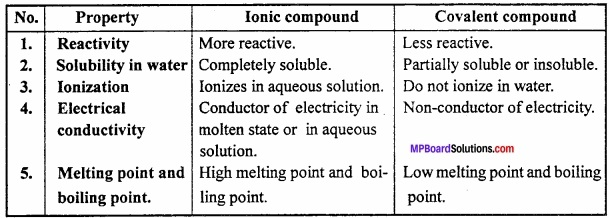Question 4.
On what factors the formation of ionic bond depends?
Factors influencing Ionic Bond formation:
The formation of ionic bond (Electrostatic force of attraction) depends upon the following factors:
1. Ionisation enthalpy:
One of the combining atoms (metal) must have low ionisa-tion enthalpy. So that, cation formation becomes easy. For example, alkali and alkaline earth metals of periodic table has tendency to form positive ion because they have comparatively low ionisation energy.

2. Electron gain enthalpy:
The electrons released in the formation of cation are to be accepted by the other atom taking part in the ionic bond formation. The electron accepting tendencies of an atom depends upon the electron gain enthalpy. (MPBoardSolutions.com) It may be defined as: Energy released when an isolated gaseous atom takes up an electron to form an anion. Greater the negative electron gain enthalpy, easier will be the formation of anion or negative ion. The halogen present in group 17 have the maximum tendency to form anions as they have very high negative electron gain enthalpy.

3. Lattice energy:
The amount of energy released when one mole of ionic solid is formed by the close packing of its constituents. It is denoted by ∆LH and negative in nature.
A(g)+ + B(g) → A+B(s) + Lattice enthalpy (∆LH)
Thus, greater the magnitude of -ve lattice energy, more will be the stability of the ionic bond or ionic compound.Question 5.
Explain the main points of Molecular orbital theory?
The main points of Molecular orbital theory are:
1. In a molecule, electrons are present in new orbitals called molecular orbitals. These molecular orbitals are characterised by a set of quantum numbers just like atomic orbitals.

2. Molecular orbitals are formed by combination of atomic orbitals of equal energies (in case of homonuclear molecules) or of comparable energies (in case of heteronuclear molecules).

3. The number of molecular orbitals formed is equal to the number of atomic orbitals undergoing combination.

4. Two molecular orbitals are formed by combination of two atomic orbitals one of these two molecular orbitals has a lower energy and the other has a higher energy than either of the combining atomic orbitals. The molecular orbital with lower energy is called bonding molecular orbital and the other is called antibonding molecular orbital.

Question 6.
What is resonance? Explain with example?
When properties of a molecule are not explained by one structure and two or more than two structures are assigned to express its characteristics, it is said that molecule is resonance hybrid of these structures and this property is known as resonance. Different resonating structures are exhibited by using sign (↔) in between these structures.
Example: Carbon dioxide (CO2) is represented by following three structures:Chemical Bonding And Molecular Structure Long Answer Type Questions – II

Question 1.
Show the molecular orbital energy levels of N2 by diagram?
N2 molecule: Each nitrogen atom contains seven electron. Thus total 14 electrons are filled in seven orbitals of increasing energy.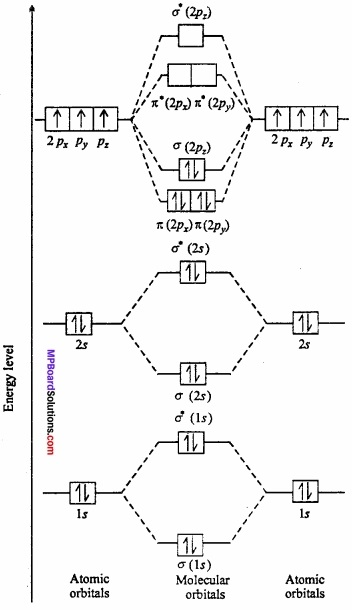Molecular orbital structure of N2 molecule will be as follows: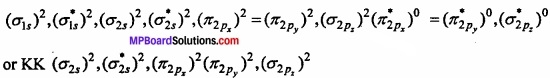Where KK represents closed shell structure (σ 1s)2($$({ \sigma _{ 1s } })^{ 2 }$$)($$({ \sigma ^{ * }_{ 1s } })^{ 2 }$$
This structure shows that it contains 10 bonding and 4 antibonding electrons.
Bond order = $$\frac{1}{2}$$ [Nb – Na] = $$\frac{1}{2}$$ [10 – 4] = 3.
Value of bond order is more. Hence value of bond energy should also be high. Experimental value of bond energy is 945 kJ mol-1 which proves the presence of paired electrons in nitrogen molecule. Thus, it is a diamagnetic molecule.

How to Calculate Bond Order. Calculations Step by Step.

Question 2.
Give the important applications of dipole moment?
Applications of Dipole moment:
1. Comparison of relative polarity:
It is possible by comparing the value of dipole moment e.g., HF (1.98 D) is more polar than HCl (1.03D).

2. Predicting the nature of molecules:
Molecules with specific dipole moments are polar in nature while those with zero value are non – polar. Thus, BeF2 (µ – 0D) is non – polar while H2O (µ = 1.84D) is polar.

3. Calculation of percentage ionic character:
% ionic character = $$\frac{Observed dipole moment}{Caluculated dipole moment}$$ × 100 (100% ionic character)
or % I.C. = $$\frac { \mu _{ obs } }{ \mu _{ cal } }$$ × 100
For example, the observed dipole moment of HCl molecule is 1.03D. For 100% ionic character i.e., complete transfer of electron charge on H+ and Cl ions would be equal to one unit (4.8 × 10-10e.s.u.)× (1.275 × 10-8 each. The bond length of H – Cl bond is 1.275 × 10-8cm. Therefore, dipole moment for complete electron transfer
µ = q × d = (4.8 × 10-10 e.s.u) × (1.275 × 10-8cm)
= 6.12 × 10-18 e.s.u cm = 6.12D
Observed dipole moment, µ(obs) = 1.03D
% ionic character = $$\frac { 1.03 }{ 6.12 }$$ × 100 = 16.83 %

4. Dipole moment of symmetric molecules is zero, but they have two or more than two polar bonds. It is applied for the measurement of symmetry.

5. Distinction between ortho, meta and para isomers of aromatic compounds:
In general, the dipole moments follow the order: ortho > meta > para e.g., In dichlorobenzene, the dipole moments of o, m and p isomers are: 2.54 D, 1.48 D and 0 respectively.Question 3.
What is valence shell electron pair repulsion theory? Write its limitations? Or, Explain valence shell electron pair repulsion theory with example?
VSEPR theory:
On the basis of this theory, “When central atom is surrounded by only bonded electron pairs, in that case the geometry of the molecule will be ordinary, but if the central atom is surrounded by bonded electron pairs as well as lone pairs or non – bonded pairs, then the geometry of die molecule will become abnormal.” This (MPBoardSolutions.com) means that the repulsion between the non – bonded or lone pair of electrons and bonded electron pairs become greater than that of repulsion between only bonded electron pairs. Repulsion

Between the electron pairs is in the following order:
lone pair – lone pair > lone pair – bonded pair > bonded pair – bonded pair

Shapes of some molecules accroding to VSEPR theory:
1. Shape of CH4:
In methane, central atom carbon is surrounded by four electron pairs and four C – H bonds. This molecule has tetrahedral geometry. Shared electrons are at the comers of tetrahedron for maximum separation. Bond angle is 109°28′.2. Shape of H2O:
In water molecule central oxygen atom is surrounded by four electron pairs, two of which are lone pair of electrons. Thus, Ip – lp and lp – bp repulsion exist. Due to this, bond angle reduces to 104.5° in place of 109°28′ and shape becomes V shaped.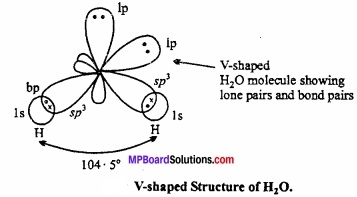3. Shape of NH3:
In ammonia, central N atom is surrounded by four electron pairs, so shape of molecule is tetrahedral. According to VSEPR theory, the four groups around the central atom of ammonia should be tetrahedrally arranged at bond angle of 109°28’.

But, the measured bond angle is 107°. This is explained on the basis of repulsive effect of the lone pair of electrons on bonding electrons. In ammonia molecule there is a lone pair of electrons on the N atom. Thus, the shape of NH3 molecule is distorted and it looks like pyramidal and it is polar in nature.Example: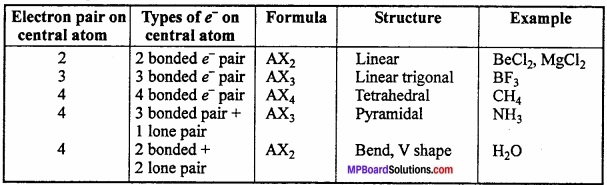Limitations of VSEPR theory:
VSEPR theory no doubt, theoretically gives the shapes of simple molecules but could not explain them and also has limited application. To overcome these limitations, two important theories based on quantum mechanical principles are commonly used. These are:

1. Valence bond theory (VBT) and
2. Molecular orbital theory (MOt).

Question 4.
Structure of two molecules are given:

1. Among these which contain intermolecular hydrogen bond and intramolecular hydrogen bond?
2. The melting point of any compound depends upon hydrogen bonding also, on the basis of this explain which one have high M.P.
3. The solubility of any compound depends upon its tendency to formed H – bonding with water among these who will form H – bond with water easily.1. Compound (a) forms intramolecular H – bond. When H – bond is present between the atoms of same molecule than it is called intramolecular H – bond.
In ortho nitrophenol [(a)] H – bond is present between two O – atoms.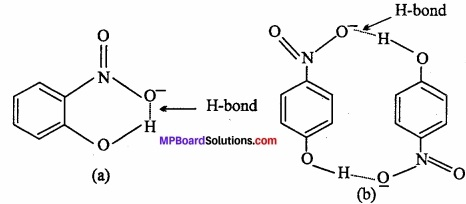In compound (b) inter molecular H – bond forms.
In p – nitrophenol [(b)] between – N02 and – OH vacant space is present. So among H – atom of one molecule and O – atom of another molecule, H – bond is formed.

2. The melting point of compound (b) is high because many molecules are forming H – bond.

3. Due to intramolecular H – bonding, compound (a) will not form H – bond with water so it is less soluble in water. Whereas compound (b) forms H – bond easily with water so soluble in water.Question 5.
Draw molecular orbital diagram for 02 molecule?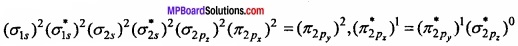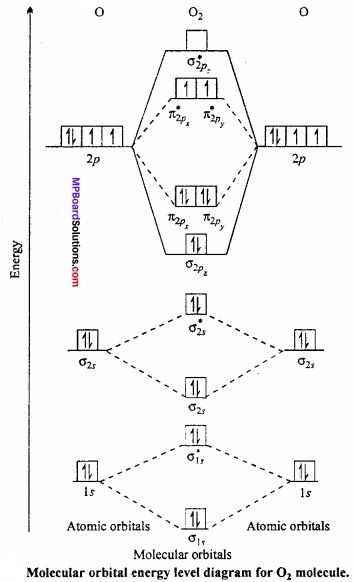∴ Bond order = $$\frac{1}{2}$$ [Nb – Na] = $$\frac{1}{2}$$ [10 – 6] = 2